#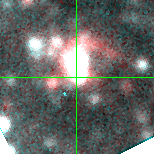M33C-21336 (J013406.83+304722.4)

## Target Notes### Classification: HII

Unresolved blend with J013406.81+304722.1 1 arcsecond away about 1 magnitude fainer in V (comparable brightness in BRI). Resolved from J013406.80+304727.0 of comparable brightness 4 arcseconds N.

## Plots of Brightness and Color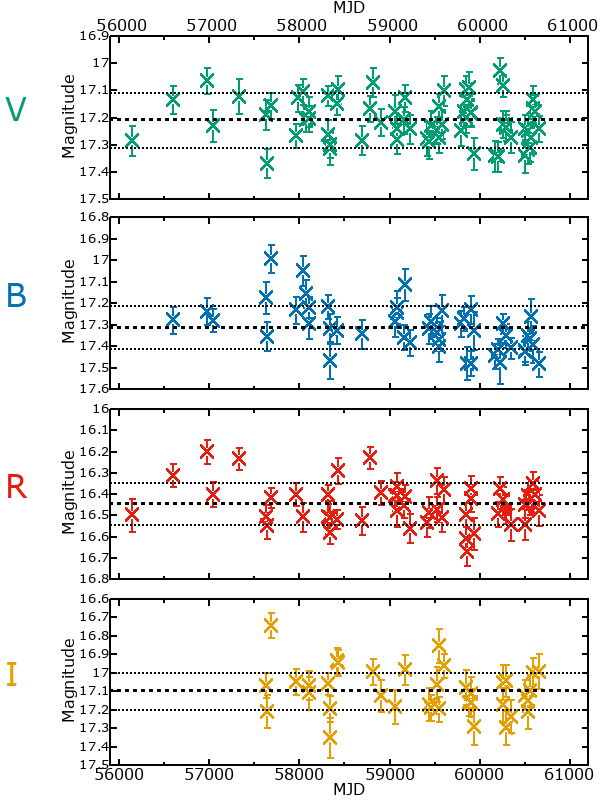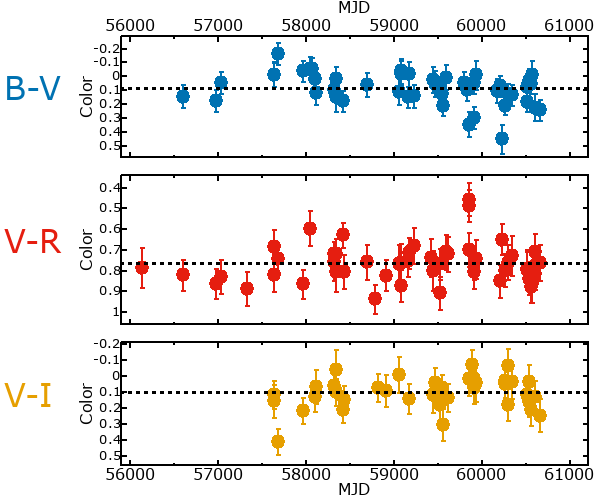## Variability

From the data available in this survey, it is not possible to tell with certainty if this star is variable.

### Correlation factors between bands

If the brightness variation is true variability then there should be a correlation between brightness changes recorded in different filter bands. The following table are the R-squared linear correlation factors calculated for the pairs of bands. The number in parentheses next to the R-squared value is the number of elements used to calculate the correlation factor. The number in square brackets next to the band is the range of magnitudes measured.

V [0.53 mag]B [0.43 mag]R [0.39 mag]
B [0.53 mag]0.21 (22)
R [0.39 mag]0.14 (25)0.07 (20)
I [0.74 mag]0.20 (15)0.07 (11)0.27 (12)

### Magnitude-Magnitude Plots

The plots below are provided to check the values in the table above and also to show any non-linear correlations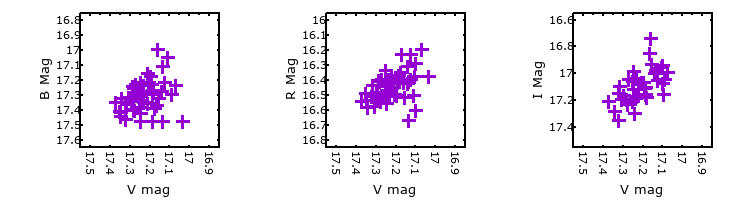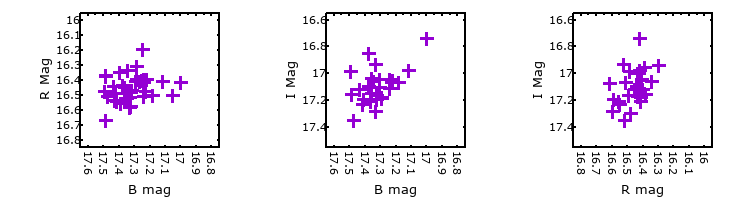### I & R Statistics

Welch & Stetson (1993, AJ, 105, 1813) present a method for varible star detection in CCD images from automated surveys. Data from two bands are compared and two statistics are calculated: R and I.

The I-statistic has an expectation value of zero for stars that are NOT variable. A larger non-zero I-value indicates a higher likelihood that the star is variable. The R statistic indicates how the color of the star changes as the variable grows brighter with R = 1.0 being no change in color.

R and I values have only been calculated if there are more than four (4) matched measurements of brightness in each band.
V
(I|R)
B
(I|R)
R
(I|R)
B
(I|R)
1.20 | 0.94
R
(I|R)
0.84 | 0.942.01 | 0.11
I
(I|R)
1.90 | 1.000.56 | 0.871.64 | 0.77